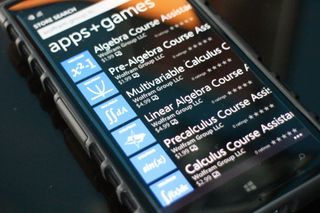# Wolfram launches seven course assistant apps on Windows Phone to turn you into a math whizAfter officially launching WolframAlpha for Windows Phone and Windows 8.1 earlier this year, Wolfram is now providing seven new course assistant apps. The apps -- which are powered by the WolframAlpha computational knowledge engine -- are designed to assist you in solving math problems and learning new concepts with ease.

## Algebra Course Assistant

First up is the Algebra Course Assistant, which covers topics found in Algebra I, Algebra II, and College Algebra:

• Evaluate any numeric expression or substitute a value for a variable.
• Simplify fractions, square roots, or any other expression.
• Solve a simple equation or a system of equations for specific variables.
• Plot basic, parametric, or polar plots of the function(s) of your choice.
• Expand any polynomial.
• Factor numeric expressions, polynomials, and symbolic expressions.
• Divide any two expressions.
• Find the partial fraction decomposition of rational expressions.

As with all Wolfram apps, Algebra Course Assistant is universal (buy it once and use on both Windows and Windows Phone), and is available now for \$1.99.

## Calculus Course Assistant

For students taking calculus courses, the Calculus Course Assistant is bound to come in handy. The \$2.99 app aims to teach you concepts from Calculus, AP Calculus AB, AP Calculus BC, Calculus I, and Calculus II:

• Evaluate any numeric expression or substitute a value for a variable.
• Plot basic, parametric, or polar plots of the function(s) of your choice.
• Determine the limit of a function as it approaches a specific value.
• Differentiate any function or implicit function.
• Find the critical points and inflection points of a function.
• Identify the local and absolute extrema of a function.
• Integrate a function, with or without limits.
• Sum a function given a lower and upper bound.
• Find the closed form of a sequence or generate terms for a specific sequence.

Here are the links for Calculus Course Assistant:

## Multivariable Calculus Course Assistant

If you're taking Multivariable Calculus, Advanced Calculus, or Vector Calculus, look no further than the Multivariable Calculus Course Assistant, which lets you:

• Evaluate any numeric expression, or substitute a value for a variable
• Plot 2D or 3D functions of your choice
• Determine the limit of a function as it approaches a specific value or values
• Differentiate any single or multivariable function
• Find the critical points and saddle points of a function
• Calculate the gradient of a function
• Identify the local extrema of a function
• Find the single, double, or triple integral of a function
• Determine the dot or cross product of two vectors
• Calculate the divergence or curl of a vector field

## Linear Algebra Course Assistant

Need to solve a system of equations, or work with matrices (learning about eigenvectors can be quite challenging) in linear algebra? Linear Algebra Course Assistant is here to guide you:

• Solve an equation or a system of equations
• Add or subtract any two vectors
• Find the cross product or dot product for two vectors
• Find the dimensions, transpose, adjugate, rank, inverse, determinant, and reduced row echelon form of a matrix
• Calculate a matrix product
• Compute linear transformations
• Determine subspaces, including row space, column space, and null space
• Find the characteristic equation, eigenvalues, and eigenvectors of a matrix

## Pre-Algebra Course Assistant

For those taking pre-algebra courses, the Pre-Algebra Course Assistant is here:

• Find the divisors and prime factorization of a number
• Calculate the GCD and LCM of two numbers
• Determine the percent change
• Reduce and round numbers
• Evaluate expressions
• Solve equations and simplify expressions
• Convert units of length, area, volume, and weight
• Compute the mean, median, and mode of a dataset
• Plot equations on the coordinate plane
• Graph inequalities on a number line
• Calculate the area, surface area, or volume of a geometric figure
• Find the midpoint, slope, and distance between two points

## Precalculus Course Assistant

Wolfram is also offering a course assistant for students taking precalculus classes. The \$1.99 Precalculus Course Assistant app allows you to:

• Evaluate any numeric expression or substitute a value for a variable
• Solve a single equation or a system of equations
• Plot functions on the x-y plane or draw a parametric or polar plot
• Determine the sine, cosine, and tangent of a specific angle in a right triangle
• Simplify, expand, or factor trigonometric functions
• Find the partial fraction decomposition of an expression
• Calculate the dot product, cross product, and magnitude of two vectors
• Identify the mean, median, mode, and standard deviation of a set of data
• Calculate permutations and combinations

## Statistics Course Assistant

And finally, if you're keen on finding out the chances of Arsenal winning something this year, you should take a statistics course. And then buy Statistics Course Assistant to guide you along the way. Here's what you get for \$1.99:

• Create a bar chart, histogram, or scatter plot of any set of data
• Find the mean, median, mode, standard deviation, quartiles, and interquartile range of a dataset
• Calculate normal probabilities and find information about the normal distribution
• Calculate binomial probabilities and find information about the binomial distribution
• Compute probabilities based on dice rolls and coin flips
• Find the best-fit line of a set of data points
• Select random integers or random real numbers